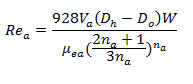## Reynold Number for Drilling Hydraulics

Reynold number is the important figure because it demonstrates the flow regimes of drilling mud as laminar, transition or turbulent flow in annulus of the wellbore. In order to correctly calculate the Reynolds number, you need to use the effective viscosity, µea, which we already discuss about it from the previous topic.

The following equation is for Reynolds number in the annulus.Where:

Rea = Reynold Number in the annulus

Va = Annular velocity, ft/min

Dh = Diameter of wellbore, inch

Do = Outside Diameter of tubular, inch

W = mud weight, ppg

µea = effective viscosity in the annulus, centi-poise

na = power law constant